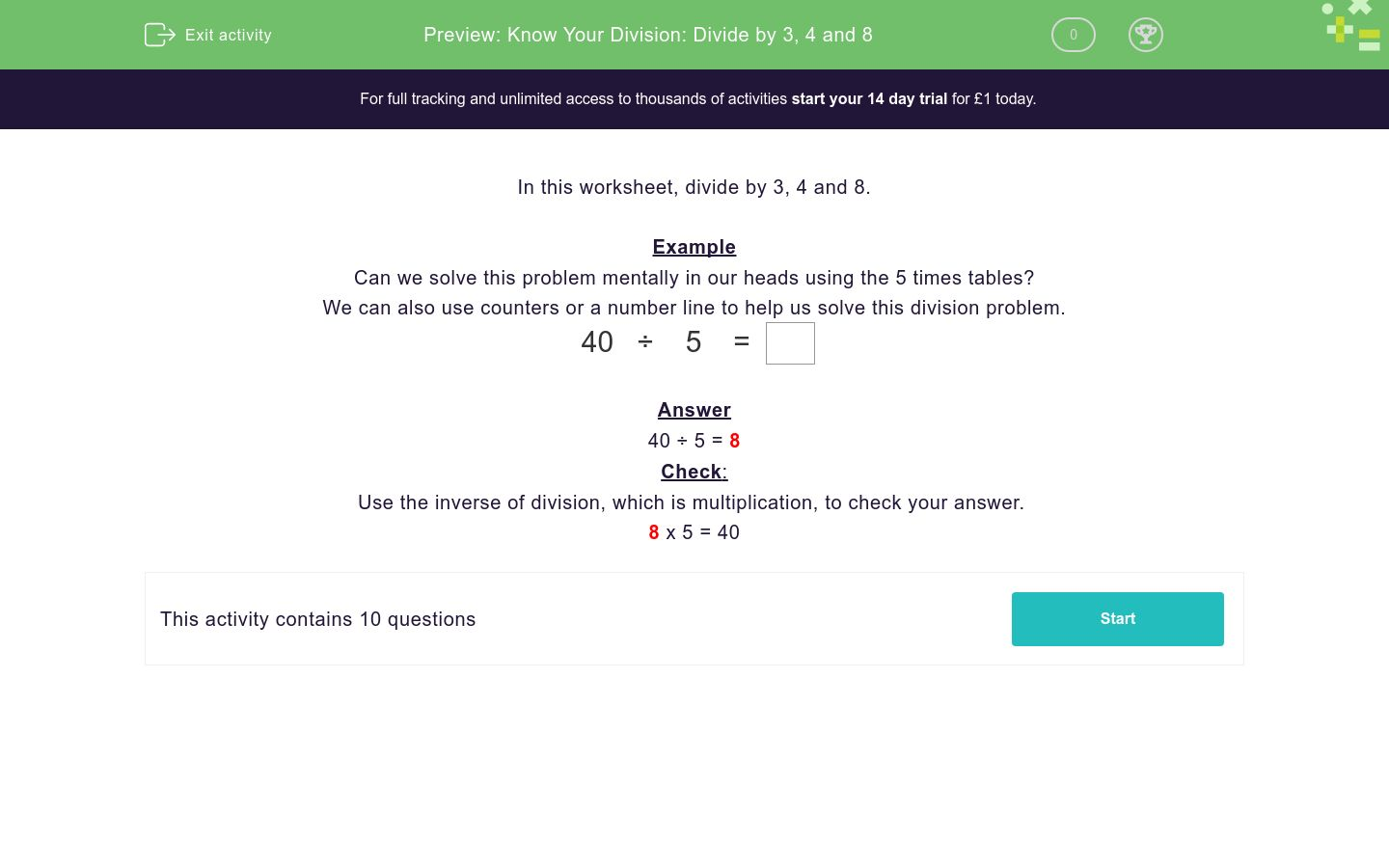# Know Your Division: Divide by 3, 4 and 8

In this worksheet, students divide by 3, 4 and 8.Key stage:  KS 2

Curriculum topic:   Number: Multiplication and Division

Curriculum subtopic:   Use Multiplication/Division Facts (3, 4 and 8)

Difficulty level:### QUESTION 1 of 10

In this worksheet, divide by 3, 4 and 8.

Example

Can we solve this problem mentally in our heads using the 5 times tables?

We can also use counters or a number line to help us solve this division problem.

 40 ÷ 5 =

40 ÷ 5 = 8

Check:

Use the inverse of division, which is multiplication, to check your answer.

8 x 5 = 40

Solve mentally, with counters or with a number line.

 27 ÷ 3 =

Solve mentally, with counters or with a number line.

 24 ÷ 4 =

Solve mentally, with counters or with a number line.

 16 ÷ 4 =

Solve mentally, with counters or with a number line.

 32 ÷ 8 =

Solve mentally, with counters or with a number line.

 21 ÷ 3 =

Solve mentally, with counters or with a number line.

 44 ÷ 4 =

Solve mentally, with counters or with a number line.

 40 ÷ 8 =

Solve mentally, with counters or with a number line.

 18 ÷ 3 =

Solve mentally, with counters or with a number line.

 3 ÷ 3 =

Solve mentally, with counters or with a number line.

 48 ÷ 8 =
• Question 1

Solve mentally, with counters or with a number line.

 27 ÷ 3 =
9
EDDIE SAYS

27 ÷ 3 = 9

3 x 9 = 27

• Question 2

Solve mentally, with counters or with a number line.

 24 ÷ 4 =
6
EDDIE SAYS

24 ÷ 4 = 6

6 x 4 = 24

• Question 3

Solve mentally, with counters or with a number line.

 16 ÷ 4 =
4
EDDIE SAYS

16 ÷ 4 = 4

4 x 4 = 16

• Question 4

Solve mentally, with counters or with a number line.

 32 ÷ 8 =
4
EDDIE SAYS

32 ÷ 8 = 4

4 x 8 = 32

• Question 5

Solve mentally, with counters or with a number line.

 21 ÷ 3 =
7
EDDIE SAYS

21 ÷ 3 = 7

3 x 7 = 21

• Question 6

Solve mentally, with counters or with a number line.

 44 ÷ 4 =
11
EDDIE SAYS

44 ÷ 4 = 11

4 x 11 = 44

• Question 7

Solve mentally, with counters or with a number line.

 40 ÷ 8 =
5
EDDIE SAYS

40 ÷ 5 = 8

5 x 8 = 40

• Question 8

Solve mentally, with counters or with a number line.

 18 ÷ 3 =
6
EDDIE SAYS

18 ÷ 3 = 6

3 x 6 = 18

• Question 9

Solve mentally, with counters or with a number line.

 3 ÷ 3 =
1
EDDIE SAYS

3 ÷ 3 = 1

3 x 1 = 3

• Question 10

Solve mentally, with counters or with a number line.

 48 ÷ 8 =
6
EDDIE SAYS

48 ÷ 8 = 6

6 x 8 = 48

---- OR ----

Sign up for a £1 trial so you can track and measure your child's progress on this activity.

### What is EdPlace?

We're your National Curriculum aligned online education content provider helping each child succeed in English, maths and science from year 1 to GCSE. With an EdPlace account you’ll be able to track and measure progress, helping each child achieve their best. We build confidence and attainment by personalising each child’s learning at a level that suits them.

Get started